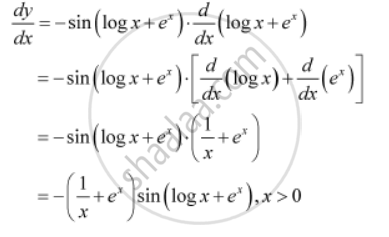Share

# Differentiate the Following W.R.T. X: Cos (Log X + Ex), X > 0 - CBSE (Commerce) Class 12 - Mathematics

ConceptExponential and Logarithmic Functions

#### Question

Differentiate the following w.r.t. x:

cos (log x + ex), x > 0

#### Solution

Let y = cos (log x + e^x)

By using the chain rule, we obtainIs there an error in this question or solution?

#### APPEARS IN

NCERT Solution for Mathematics Textbook for Class 12 (2018 to Current)
Chapter 5: Continuity and Differentiability
Q: 10 | Page no. 174

#### Video TutorialsVIEW ALL 

Solution Differentiate the Following W.R.T. X: Cos (Log X + Ex), X > 0 Concept: Exponential and Logarithmic Functions.
S